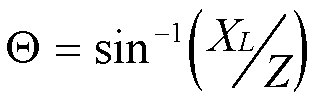## Introduction to Eddy Current Technology,

Historical backgraound and Electrical Theory

This document is intended as a brief familiarisation course for Hocking NDT's customers and sales representatives. It is not intended as a complete theoretical course in Eddy Current Non destructive testing and a number of aspects are intentionally simplified. The bibliography at the end of the document details several appropriate reference books which should be consulted if a more complete understanding is required

### Historical Context

Eddy current testing has its origins with Michael Faradays discovery of electromagnetic induction in 1831. In 1879 Hughes recorded changes in the properties of a coil when placed in contact with metals of different conductivity and permeability, but it was not until the second world war that these effects were put to practical use in testing materials.

Much work was done in the 1950's and 60's, particularly in the aircraft and nuclear industries and Eddy current testing is now an accurate, widely used and well-understood inspection technique.

### Basic Electrical Theory: Current Flow in DC circuits

When a voltage is applied to a circuit containing only resistive elements current flows according to ohms law:

I = V/R or V = I x R

If a circuit consists of more than one element the overall voltages, resistance and current can be calculated by simple algebra, for example, with two resistors in series: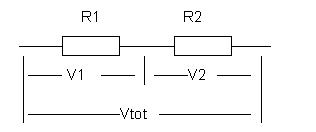Current (I) must be the same for both resistors, so:

V1 = I x R1, V2 = I x R2,

Vtot = V1+V2 = I x R1 + I x R2 = I x (R1+R2) = I x Rtot

so Rtot = R1+ R2

### Electromagnetic Induction

In 1824 Oersted discovered that current passing though a coil created a magnetic field capable of shifting a compass needle. Seven years later Faraday and Henry discovered the opposite; that a moving magnetic field would induce a voltage in an electrical conductor.-

The two effects can be shown in a simple transformer connected to a DC supply as below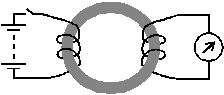The meter needle will kick one way when current is applied then back the other way when it is removed. - A voltage is only induced when the magnetic field is changing

Such a voltage is also induced in the first winding, and will tend to oppose the change in the applied voltage.

The induced voltage is proportional to the rate of change of current.

A property of the coil called inductance (L) is defined, such that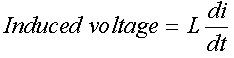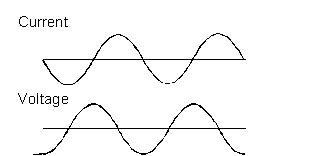If an AC current flows though an inductor the voltage across the inductor will be a maximum when the rate of change of current is greatest . For a sinusoidal wave form this is at the point where the actual current is zero,

Thus the voltage applied to an inductor reaches its maximum value a quarter-cycle before the current does - the voltage is said to lead the current by 90 degrees

The value of the voltage and current can be calculated from the formula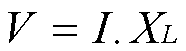where XL is the inductive reactance, defined by the formula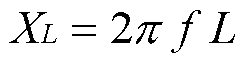where f is the frequency in Hz

### Current flow in AC Circuits - Impedance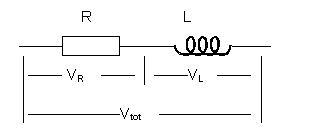As we saw above, for series DC circuits calculation of total resistance is simply a matter of adding the individual resistance values.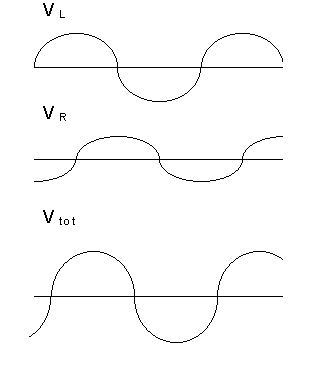For an AC circuit things are not so simple, but the same basic principles apply:

The current though both elements must be the same, and at any instant the total voltage across the circuit is the sum of the values across the elements, however the maximum voltage across the resistance coincides with zero voltage across the inductor and vice versa.

We can represent this graphically using a Vector Diagram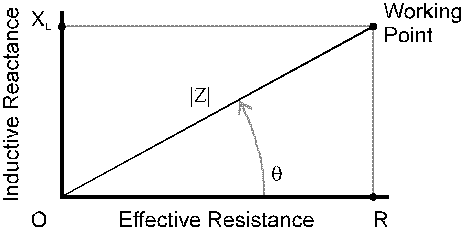The Impedance of the circuit is therefore given by the formula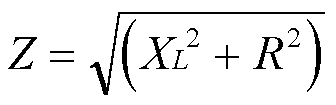and the phase angle between voltage and current is given by